# Power compensations in prescription dive masks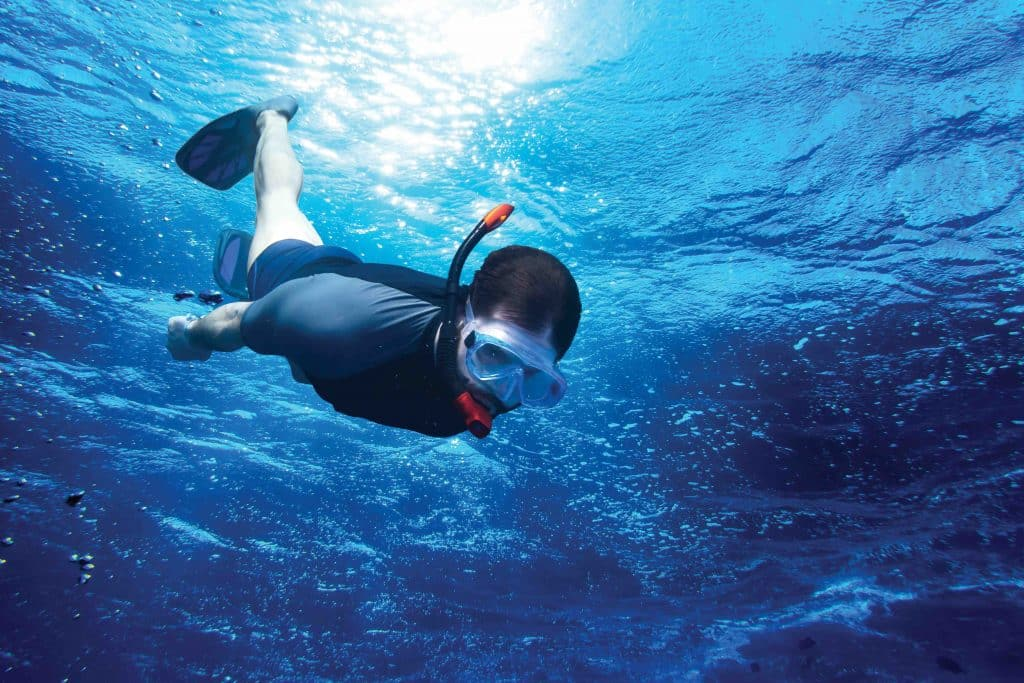A common misconception when glazing dive masks is that the refractive index of water needs to be compensated for. GRANT HANNAFORD demonstrates why this is not quite the case.

One of the more common sources of confusion that appears on forums is related to the application of prescription to diving masks. This arises from the immersion of the appliance in water and attempts to compensate for the different refractive index of water as compared to air.

The power of a surface is defined by the following expression: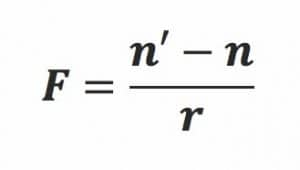For a front surface, with F denoting power, n’ = second medium (ie the one into which light is travelling), n = first medium (the one from which light is travelling) and r = radius of curvature.

In terms of front and back surfaces of a lens this becomes: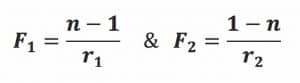With n=1 being the refractive index for air. With our dive mask problem we no longer have the refractive index of the surrounding medium as n=1, so we would expect the power of the surface, and subsequently the entire optical system, to change when compared to immersion in air.

Following are the same surfaces (r=0.1m) for a CR39 lens (n=1.498) in air (n=1) and water (n=1.333).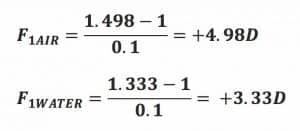Immersion of the surface in water has reduced the surface power, as the difference between the refractive index of the two media has decreased. Snell’s Law is, in part, the ratio between the refractive index of the two media, so we can see why this occurs.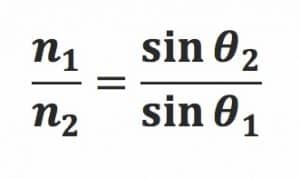This extends to the refracting power of the cornea when immersed in water.

When we open our eyes under water the power of the cornea is reduced, impairing visual performance. Using the relationship between the refractive indices of our media we arrive at the following expression: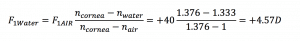Which is a loss of corneal power of over 88% when the eye is immersed in water.

The reduction is attenuated somewhat overall in the visual system, but the influence of immersion must be overcome to attain clear vision underwater. The simplest solution is to maintain the index of the medium surrounding the cornea at n=1, hence the use of a goggle to create an air pocket around the eyes.

Why doesn’t the immersion in water influence the surface of the goggle in the same way as it does the cornea?

Simply, the surface of the goggle lenses are plano so the variance in refractive indices has no effect: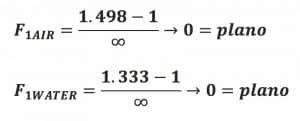As the limit of 1/x as x approaches infinity is zero, which is true for both cases.

In practice we have observed that there needs to be some measure of compensation when applying prescriptions to goggles, especially dive masks.

Anecdotally, a reduction of about 33% of lens power is quoted for dive masks. This may be attributed to the difference between the refractive index of air and water, ie: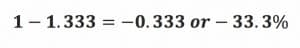This approach over-compensates by a large amount. While it seems intuitive, it does not account for the relationship between the refractive indices and the refracting media being paramount, not just the relationship between immersing media.

The source of the need to compensate powers is, once again, quite simple. If we measure the vertex distance for dive masks the vertex distances will tend to around 50mm, as opposed to the typical values of 12mm for spectacles.

We can therefore work out the variance in vertex distances and compensate for the increased distance to the back surface of the lens as mounted in the goggle.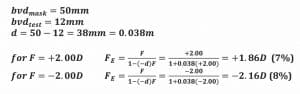As this relationship is non linear, the simplest way is to calculate these values or use one of the available compensation charts. Similarly the creation of a spreadsheet is a simple matter that allows for more customisation.

The entire purpose of goggles and dive masks is to place the cornea in air, negating the impact of immersion in a medium with an index other than n=1. Because of this it is important when working with dive masks to identify the arrangement of lenses with relation to the environment.

If the front surface of the lens immersed in water is anything other than plano then power compensations will need to be made to the optical system in addition the normal vertex distance compensations.

For the most common dive mask designs with a plano front surface the primary compensation will be due to the larger vertex distance, not the immersion in water.

This is a summary of an article available at www.aaoo.net.au which contains full derivations and tables supporting the content above.